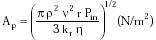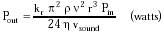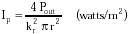Nanomedicine, Volume I: Basic Capabilities

Robert A. Freitas Jr., Nanomedicine, Volume I: Basic Capabilities, Landes Bioscience, Georgetown, TX, 1999

The acoustic power generated by any vibrating source is Pout = U2 RA (RMS watts), where U is the rate of oscillatory volume displacement of the fluid, measured in RMS m3/sec, and RA is the acoustic radiation resistance seen by the source, measured in MKS acoustic ohms.889 Two basic generators are the vibrating piston and the pulsating sphere, both of radius r. From Stokes Law (Eqn. 9.73) the input power required to propel a circular piston through water at velocity v is Pin ~ 6 p r h v2, or Pin ~ 24 p r h v2 for a radially oscillating sphere. Hence U = p r2 v = (p r3 Pin / 6 h)1/2 for a vibrating piston to which Pin watts of mechanical input power are delivered, and U = (2 p r3 Pin / 3 h)1/2 for the pulsating sphere.

The radiation resistance RA = p r n2 / kr vsound, where kr = 2 (piston) or 4 (sphere) and n is acoustic frequency (Hz). This equation is valid only when r/l << 1 (e.g., radiator size is very small in comparison to acoustic wavelength l = vsound / n); for r = 1 micron and n = 1 MHz, then r/l ~ 0.001 in water. Sound energy generated from a source with dimensions small compared with the wavelength of the vibration in the medium produces an intensity uniform in all angular directions; such a generator is considered to be a point source. Formulas for radiators having r/l >> 1 are also provided by Massa.889

Combining these results with Eqn. 4.53, transmitted acoustic pressure (Ap), output power (Pout), and power intensity (Ip) at the radiator surface are given by{Eqn. 7.6}{Eqn. 7.7}{Eqn. 7.8}

For example, a vibrating piston radiator of radius r = 1 micron and input power Pin = 10 pW operating at n = 1 MHz in vivo produces Ap = 0.0007 atm radiation pressure, Pout = 0.005 pW (giving e% = Pout / Pin = 0.0005 (0.05%) and an acoustic intensity of Ip = 0.002 watts/m2 at the surface of the radiator, assuming r = 993.4 kg/m3, h = 1.1 x 10-3 kg/m-sec and vsound ~ 1500 m/sec for human interstitial fluid at 310 K. Figure 7.1 summarizes Eqn. 7.6 for various parameter choices.

Last updated on 19 February 2003